# Sort Positive Numbers and Negative Numbers by Absolute Values

If both positive numbers and negative numbers exist in the same column, when sorting them by absolute values, we can sort them with the help of ABS function and helper column. In this article, we will show you the way to sort numbers by absolute values.

## EXAMPLE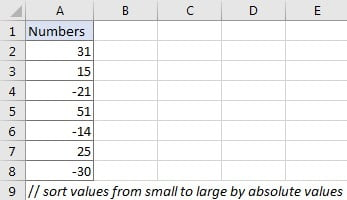We want to sort numbers in “Numbers” column from smallest to largest by absolute values.

## ANALYSIS

If we directly click “Sort Smallest to Largest” button, numbers are sorted by actual values, negative numbers are always the smallest ones. So, if we want to sort them by absolute values, we need to do below two steps:

a. Convert negative numbers to positive number.

b. Sort all positive numbers.

We can apply ABS function to help us solve this problem.

## STEPS

First of all, create a helper column. A helper column can help us save temporary data or simplifies some complex formulas. In this instance, we create a helper column to save absolute values of the given numbers.

a. In this instance, just create the helper column adjacent to “Numbers” column.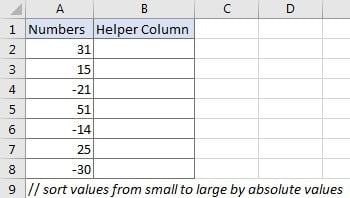b. Enter =ABS(A2) into B3 and press Enter. ABS function is used for returning the absolute value of the given number. The syntax is ABS(number).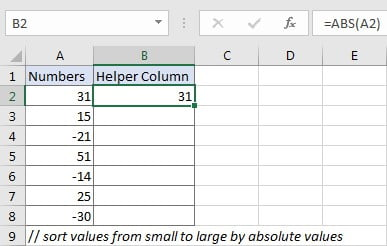c. Drag down the fill handle to copy down this formula from B2 to B8.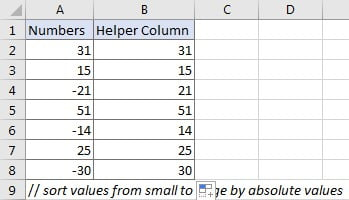d. Select range B2:B8, then click Data > Sort A to Z (Sort Smallest to Largest) in Sort & Filter section.e . Verify that below “Sort Warning” dialog pops up immediately. As we want to sort numbers in “Numbers” column (A column) with the help of helper column (B column), so just keep default option checked, and click “Sort”. If we just want to sort current selection, check on “Continue with the current selection”. The two options are mutually exclusive.f. After clicking “Sort” button on “Sort Warning” dialog, numbers are sorted from smallest to largest properly in both two columns. We can see that in A column negative numbers are still displayed with negative sign.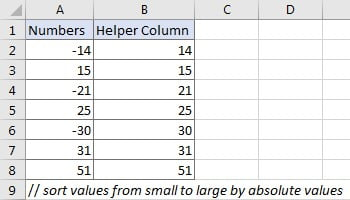g. Delete helper column, now numbers in A column are sorted by absolute values properly as we expect.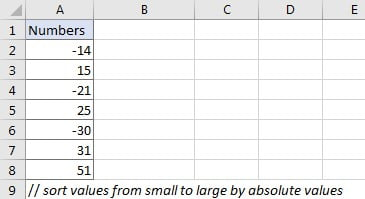### Related Functions

• Excel ABS Function
The Excel ABS function returns the absolute value of a number.The ABS function is a build-in function in Microsoft Excel and it is categorized as a Math and Trigonometry Function.The syntax of the ABS function is as below:=ABS (number)…
Related Posts

Break ties with helper COUNTIF and column

Suppose you got a task to adjust the values that contain the ties; what would be your first attempt to break the ties of the given value? If you are wondering about doing this task manually, let me add that ...

Calculate Total Cost with Excel VLOOKUP Function

In today's article we will show you how to calculate the total cost for a given weight using the Excel VLOOKUP function. This function will help us to find the appropriate unit price for that weight and then we can ...

Excel Formulas To Calculate The Bond Valuation

Assume that you've been assigned a task of calculating bond valuation; so if you are new to Ms Excel or do not have much experience with it, then I am pretty sure about it that doing this task manually might ...

Calculate Cumulative Totals with Excel SUM Function

Today, through a simple example, we will show you how to use one of the most common-used Mathematical functions in excel, the SUM function, to add up the sum. In our daily life, we keep an account of what we ...

BMI Calculation Formula In Ms Excel

You might have come across a task in which you were assigned to make BMI calculations of the supplied numbers, and you may be looking for an efficient approach to accomplish this process rather than doing BMI calculations manually, by ...

Calculate The Period of Loan or Investment in Excel

In our daily life, most of us will invest or take a loan, so we need to master some simple financial functions to calculate the period needed for loans or investments. If you work as an accountant, you need to ...

Working Time Calculation Based on Timesheets

In the office, a special machine record the time when you start working (clock in office) and when you finish working (clock out of office). We can calculate the total working time by subtracting the end working time from the ...

Sort/Rank Numeric Values with Duplicate Values Exist

Excel built-in RANK function can sort a set of values. If there are duplicate numbers, then the rank number is also duplicated. See the following example: There are two numbers “100” in range A2:A9, they are both the third largest ...

Calculate Days Open in Excel

If you want to know how to Calculate days in Excel, there are some formulas that you can use to do so. For example, you can use the DAYS function in Excel to find the number of days between two ...

Calculate Grades With VLookup in Excel

Why Should You Calculate Grades With VLookup Excel? If you're looking for a simple way to find out a student's grades, VLOOKUP Excel can do the trick. This function uses a lookup table to find the values and sort them ...

Sidebar